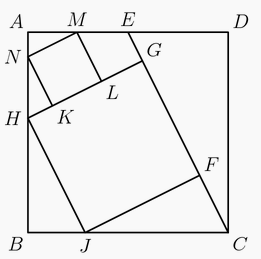###### back to index | new

The nine delegates to the Economic Cooperation Conference include $2$ officials from Mexico, $3$ officials from Canada, and $4$ officials from the United States. During the opening session, three of the delegates fall asleep. Assuming that the three sleepers were determined randomly, find the probability that exactly two of the sleepers are from the same country.

There is a prime number $p$ such that $16p+1$ is the cube of a positive integer. Find $p$.

In the diagram below, $ABCD$ is a square. Point $E$ is the midpoint of $\overline{AD}$. Points $F$ and $G$ lie on $\overline{CE}$, and $H$ and $J$ lie on $\overline{AB}$ and $\overline{BC}$, respectively, so that $FGHJ$ is a square. Points $K$ and $L$ lie on $\overline{GH}$, and $M$ and $N$ lie on $\overline{AD}$ and $\overline{AB}$, respectively, so that $KLMN$ is a square. The area of $KLMN$ is $99$. Find the area of $FGHJ$.Let $f(x)$ be a third-degree polynomial with real coefficients satisfying $$|f(1)|=|f(2)|=|f(3)|=|f(5)|=|f(6)|=|f(7)|=12.$$ Find $|f(0)|$.

Let $m$ be the least positive integer divisible by $17$ whose digits sum is $17$. Find $m$.

Two unit squares are selected at random without replacement from an $n \times n$ grid of unit squares. Find the least positive integer $n$ such that the probability that the two selected unit squares are horizontally or vertically adjacent is less than $\frac{1}{2015}$.

There are $2^{10} = 1024$ possible $10$-letter strings in which each letter is either an $A$ or a $B$. Find the number of such strings that do not have more than $3$ adjacent letters that are identical.

Let $x$ and $y$ be real numbers satisfying $x^4y^5+y^4x^5=810$ and $x^3y^6+y^3x^6=945$. Evaluate $2x^3+(xy)^3+2y^3$.

Find the number of rational numbers $r$, $0 < r < 1$, such that when $r$ is written as a fraction in lowest terms, the numerator and the denominator have a sum of $1000$.

The positive integers $N$ and $N^2$ both end in the same sequence of four digits $abcd$ when written in base 10, where digit $a$ is not zero. Find the three-digit number $abc$.

Arnold is studying the prevalence of three health risk factors, denoted by $A$, $B$, and $C$, within a population of men. For each of the three factors, the probability that a randomly selected man in the population has only this risk factor (and none of the others) is $0.1$. For any two of the three factors, the probability that a randomly selected man has exactly these two risk factors (but not the third) is $0.14$. The probability that a randomly selected man has all three risk factors, given that he has $A$ and $B$ is $\frac{1}{3}$. Find the probability that a man has none of the three risk factors given that he does not have risk factor $A$.

The repeating decimals $0.abab\overline{ab}$ and $0.abcabc\overline{abc}$ satisfy $0.abab\overline{ab}+0.abcabc\overline{abc}=\frac{33}{37},$ where $a$, $b$, and $c$ are (not necessarily distinct) digits. Find the three digit number $abc$.

Charles has two six-sided die. One of the die is fair, and the other die is biased so that it comes up six with probability $\frac{2}{3}$ and each of the other five sides has probability $\frac{1}{15}$. Charles chooses one of the two dice at random and rolls it three times. Given that the first two rolls are both sixes, find the probability that the third roll will also be a six.

Let $z$ be a complex number with $|z|=2014$. Let $P$ be the polygon in the complex plane whose vertices are $z$ and every $w$ such that $\frac{1}{z+w}=\frac{1}{z}+\frac{1}{w}$. Then the area enclosed by $P$ can be written in the form $n\sqrt{3}$, where $n$ is an integer. Find the remainder when $n$ is divided by $1000$.

Let $f(x) = x^4 + ax^3 + bx^2 + cx + d$. If $f(-1) = -1$, $f(2)=-4$, $f(-3) = -9$, and $f(4) = -16$. Find $f(1)$.

Show that, if $a,b$ are positive integers satisfying $4(ab-1)\mid (4a^2-1)$, then $a=b$

Show that $2x^2 - 5y^2 = 7$ has no integer solution.

Solve in positive integers $x^2 - 4xy + 5y^2 = 169$.

Let $b$ and $c$ be two positive integers, and $a$ be a prime number. If $a^2 + b^2 = c^2$, prove $a < b$ and $b+1=c$.

Find any positive integer solution to $x^2 - 51y^2 = 1$.

What is the remainder when $\left(8888^{2222} + 7777^{3333}\right)$ is divided by $37$?

Let positive integer $d$ is a divisor of $2n^2$, where $n$ is also a positive integer. Prove $(n^2 + d)$ cannot be a perfect square.

For any arithmetic sequence whose terms are all positive integers, show that if one term is a perfect square, this sequence must have infinite number of terms which are perfect squares.

Prove there exist infinite number of positive integer $a$ such that for any positive integer $n$, $n^4 + a$ is not a prime number.

Find that largest integer $A$ that satisfies the following property: in any permutation of the sequence $1001$, $1002$, $1003$, $\cdots$, $2000$, it is always possible to find $10$ consecutive terms whose sum is no less than $A$.